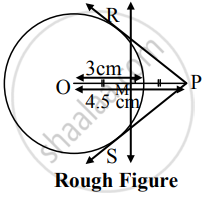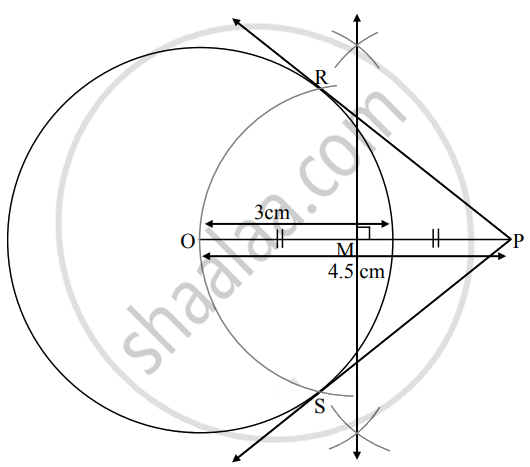# Draw a circle with center O and radius 3 cm. Take point P outside the circle such that d (O, P) = 4.5 cm. Draw tangents to the circle from point P. - Geometry

Diagram

Draw a circle with center O and radius 3 cm. Take point P outside the circle such that d (O, P) = 4.5 cm. Draw tangents to the circle from point P.

#### SolutionSteps of construction:

1. With centre O, draw a circle of radius 3 cm.
2. Take point P such that OP = 4.5 cm.
3. Draw the perpendicular bisector of seg OP. It intersects OP in point M.
4. With M as centre and radius equal to OM, draw an arc intersecting the circle in points R and S.
5. Draw rays PR and PS.
Rays PR and PS are the required tangents to the circle.
Concept: To Construct Tangents to a Circle from a Point Outside the Circle.
Is there an error in this question or solution?

Share